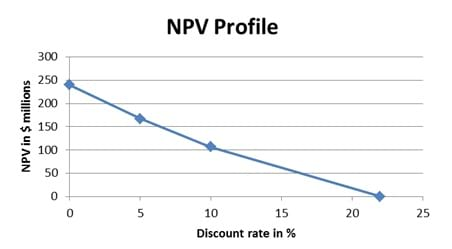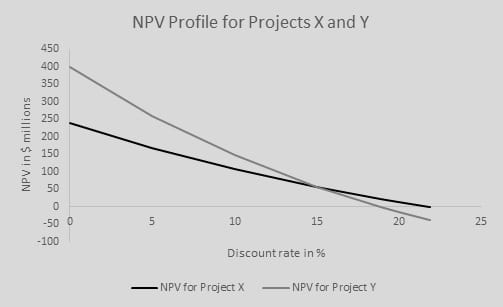IFT Notes for Level I CFA® Program
IFT Notes for Level I CFA® Program

# Part 3

### 4.3. Payback Period

The payback period is the number of years it takes to recover the initial cost of the investment.

• Easy to calculate.
• Easy to explain.
• Indicator of project liquidity. A project with two-year payback is more liquid than one with a longer payback period as the initial investment is recovered more quickly.

Drawbacks:

• Does not consider cash flows after payback period.
• It does not consider the time value of money as the cash flows are not discounted at the project’s required rate of return.
• Does not consider the risk of a project.

### 4.4. Discounted Payback Period

Discounted payback method uses the present value of the estimated cash flows; it gives the number of years to recover the initial investment in present value terms.

Drawbacks of discounted payback method:

• Does not consider any cash flows beyond the payback period.
• Poor measure of profitability as there may be negative cash flows after the discounted payback period which may result in a negative NPV.

Example 3

Compute the payback period and the discounted payback period assuming a rate of 10%.

 Year 0 1 2 3 4 Cash flows -800 340 340 340 340

Solution:

 Year 0 1 2 3 4 Cash flows -800 340 340 340 340 Cumulative Cash flows -800 -460 -120 220 560 Discounted Cash flows -800 309.1 280.99 255.45 232.22 Cumulative Discounted Cash flows -800 -490.9 -209.91 45.54 277.76

Payback period = Last year with negative cumulative cash flow + unrecovered cost at the beginning of the next year/ cash flow in the next year

Payback period == 2.35 years

Discounted payback period == 2.82 years

The discounted payback period is always going to be greater than the payback period, as long as the interest rate is positive. If the interest rate is 0%, both payback periods will be the same.

### 4.5. Average Accounting Rate of Return

The average accounting rate of return (AAR) can be defined as:

Average accounting rate of return =### 4.6. Profitability Index

Profitability Index is the present value of a project’s future cash flows divided by the initial investment.

Profitability Index PI =Profitability Index PI =Investment decision rule for PI:

Invest if PI > 1.

Do not invest if PI < 1.

Difference between PI and NPV

Consider two projects A and B. Project A has an initial investment of $1 million and an NPV of 0.1 million. Project B has an initial investment of$1 billion and an NPV of 0.2 million. If projects A and B are mutually exclusive, then project B would be chosen because of higher NPV. But, if you consider the profitability index, it gives a different picture.

PI of project A = 1 + 0.1/1 = 1.1

PI of project B = 1 + 0.2 /1000 = 1.0002

Based on PI, project A is more profitable than project B.

### 4.7. NPV Profile

NPV profile is a graph that plots a project’s NPV for different discount rates. The NPV is shown on the y-axis with the discount rates on the x-axis. Given the data below, create the NPV profile for project X.

 Year 0 1 2 3 4 Project -400 160 160 160 160

 Discount rate NPV (in \$ million) 0 240 5 167 10 107 22 0Two important points on the graph:

1. The point where the profile goes through the Y-axis (240) is the NPV of the project when the discount rate is 0. This is equal to the sum of the undiscounted cash flows.
2. The point where the profile goes through the X-axis (22) is where the discount rate is equal to the IRR of the project.

Example 4

Draw the NPV profiles for projects X and Y. Discuss the significance of crossover point.

 Year 0 1 2 3 4 Project X -400 160 160 160 160 Project Y -400 0 0 0 800

The NPV profile for projects X and Y at different discount rates is tabulated below. Based on these values, the NPV profiles are depicted graphically.

Note: The values are computed for each discount rate using the calculator.

 Discount Rate (in %) NPV for Project X NPV for Project Y 0 240 400 5 167.35 258.16 10 107.17 146.41 15 56.79 57.40 18.92 22.82 0 20 14.19 -14.19 21.86 0 -37.22

Let us plot the NPV profile for both the projects now.The point at which the NPV for both projects intersect is called the crossover point.

If X and Y are mutually exclusive, the discount rate is used to decide which project is better. At lower discount rates, i.e., to the left of the crossover point, Project Y is better. At higher discount rates, i.e., to the right of the crossover point, Project X is better. For example, at a discount rate of 10%, Project Y is better, whereas at a discount rate of 20%, Project X is better.